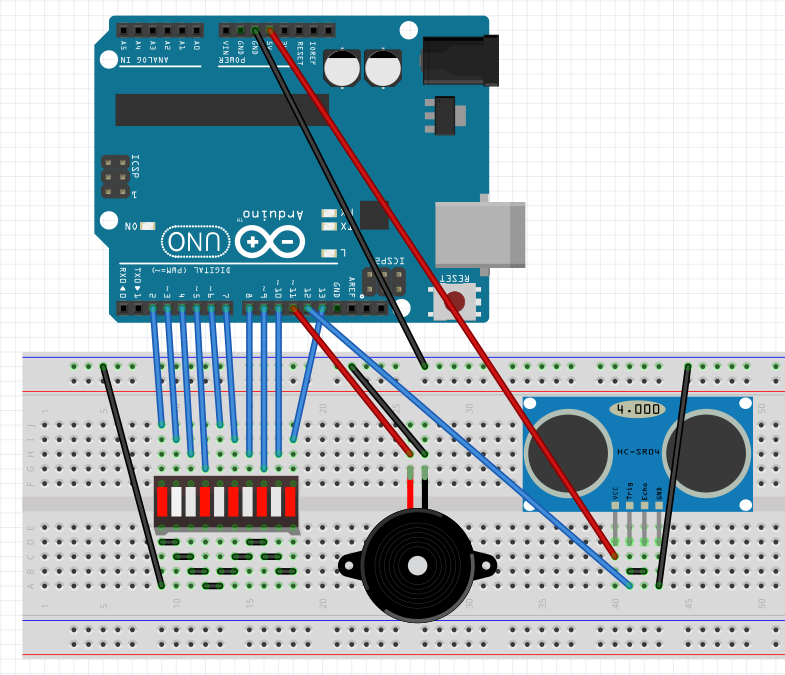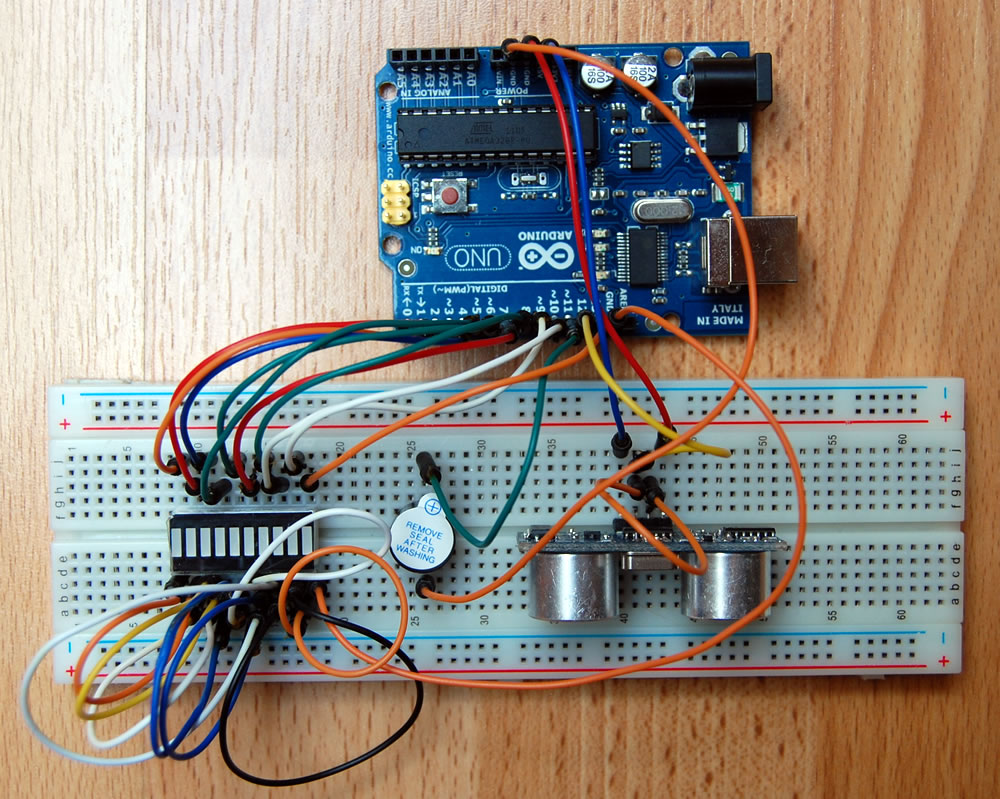# Create Object Radar with Ultrasound Distance Sensor

We use an Arduino UNO, an SR-04 ultrasound sensor, a LED bar graph and a buzzer to create an object radar that will alarm when object is closer than 10cm. The LED bar graph indicates the distance between object and the radar. When there is an object approaching to the radar, and the distance is less than 10cm, the radar starts the alarm, and the volume will increase when the object gets even closer. The LED bar graph (an array of LEDs) will indicate how close the object is.

## The Wiring

The figure below show the wiring.And here is the picture of how it is actually wired on the bread board.## Arduino Source Code

Here is the source code for the Arduino:

```const int disSensorPin = 12;
const int speakerPin = 11;
const int ledPins[] = {2, 3, 4, 5, 6, 7, 8, 9, 10, 13};
const int minLedPin = 0;
const int maxLedPin = 9;
void setup() {
for(int thisPinNum = minLedPin; thisPinNum <= maxLedPin; thisPinNum ++){
pinMode(ledPins[thisPinNum], OUTPUT);
digitalWrite(ledPins[thisPinNum], LOW);
}
pinMode(speakerPin, OUTPUT);
}
void loop() {
pinMode(disSensorPin, OUTPUT);
digitalWrite(disSensorPin, LOW);
delayMicroseconds(2);
digitalWrite(disSensorPin, HIGH);
delayMicroseconds(20);
digitalWrite(disSensorPin, LOW);
pinMode(disSensorPin, INPUT);
long time = micros();
time = micros() - time;
float distance = time*0.017;
if(distance < 100) {    // when distance is less than 100cm, light LEDs
int approaching = 100 - (int)distance;
for(int thisPinNum = minLedPin; thisPinNum <= approaching/10; thisPinNum ++) {
digitalWrite(ledPins[thisPinNum], HIGH);
if(approaching > 70) {
analogWrite(speakerPin, (approaching-70)*255/30);    // the closer, the louder
delay(20);
digitalWrite(speakerPin, LOW);
}
}
}
delay(300);
for(int thisPinNum = minLedPin; thisPinNum <= maxLedPin; thisPinNum ++) {
digitalWrite(ledPins[thisPinNum], LOW);
}
}```

## Video

Here is a short video that shows how it works: